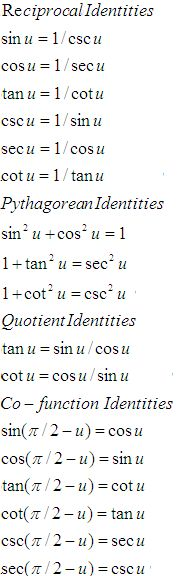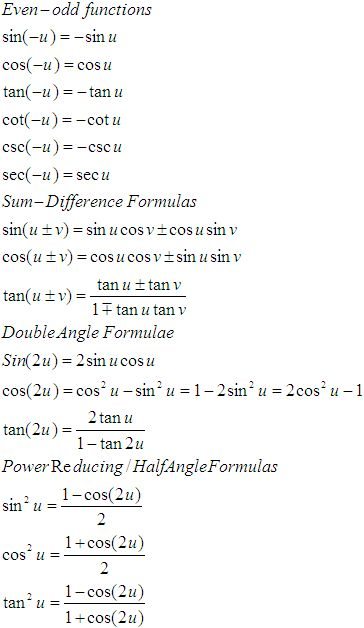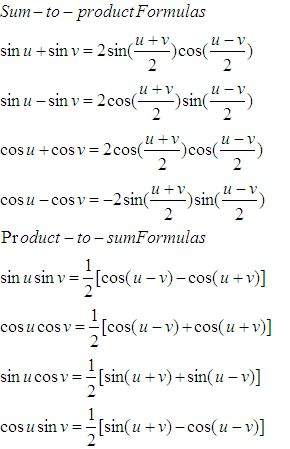Name: ___________________Date:___________________

 Email us to get an instant 20% discount on highly effective K-12 Math & English kwizNET Programs!

High School Mathematics - 210.18 Trignometry Review

 Function 0o 30° 45° 60° 90° sin Ö0/2 Ö1/2 Ö2/2 Ö3/2 Ö4/2 cos Ö4/2 Ö3/2 Ö2/2 Ö1/2 0 tan 0 Ö3/3 1 Ö3 undefined sec 1 2Ö3/3 Ö2 2 undefined csc undefined 2 Ö2 2Ö3/3 1 cot undefined Ö3 1 Ö3/3 0

 sin cos tan 0 0 1 0 90 1 0 infinity 180 0 -1 0 270 -1 0 infinityFunctions of angles in all quadrants in terms of those in quadrant 1
 -A 900 ± A P/2 ± A 1800 ± A P ± A 2700 ± A 3P/2 ± A 3600 ± A 2P ± A sin -sinA cos A ±sin A -cosA ±sin A cos cos A ±sin A -cos A ±sin A cos A tan -tan A ±cot A ± tan A ±cot A ±tan A csc -csc A sec A ± csc A -sec A ±csc A sec sec A ± csc A -sec A ±csc A sec A cot -cot A ± tan A ± cot A ±tan A ± cot A

 Q 1: In triangle ABC , if 2 cos A/a + cos B/b + 2 cos C/c = a/bc + b/ca. find angle A30 degrees45 degrees90 degrees Q 2: Find the angle in radian through which a pendulum swings if its length is 75 cm and the tip describes an arc of length 10 cm2/153/154/15 Q 3: In triangle ABC, if b = 6, c = 4, cos A = 1/12, find a431/24.31/2 Q 4: Find the angle in radian through which a pendulum swings if its length is 75 cm and the tip describes an arc of length 15 cm2/51/51/3 Q 5: A person standing on the bank of a river, observes that the angle subtended by a tree on the opposite bank is 60 degrees, when he retires 40 metres from the bank, he finds the angle to be 30 degrees. Find the height of the tree.20 metres64 metres34 metres Q 6: The elevation of a tower at a point 60 metres from it is cot-13/5 Obtain the height of the tower.110 metres45 metres100 metres Q 7: The angular elevation of a tower from a point is 30 degrees at a point in a horizontal line to the foot of the tower and 100 metres near it is 60 degrees, find the distance of the first point from the tower.Answer: Q 8: A vertical flagstaff stands on a horizontal plane from a point distant 150 metres from its foot, the angle of elevation of its top is found to be 30o, find the height of the flagstaff.100.4 metres113.4 metres100 metres Question 9: This question is available to subscribers only! Question 10: This question is available to subscribers only!